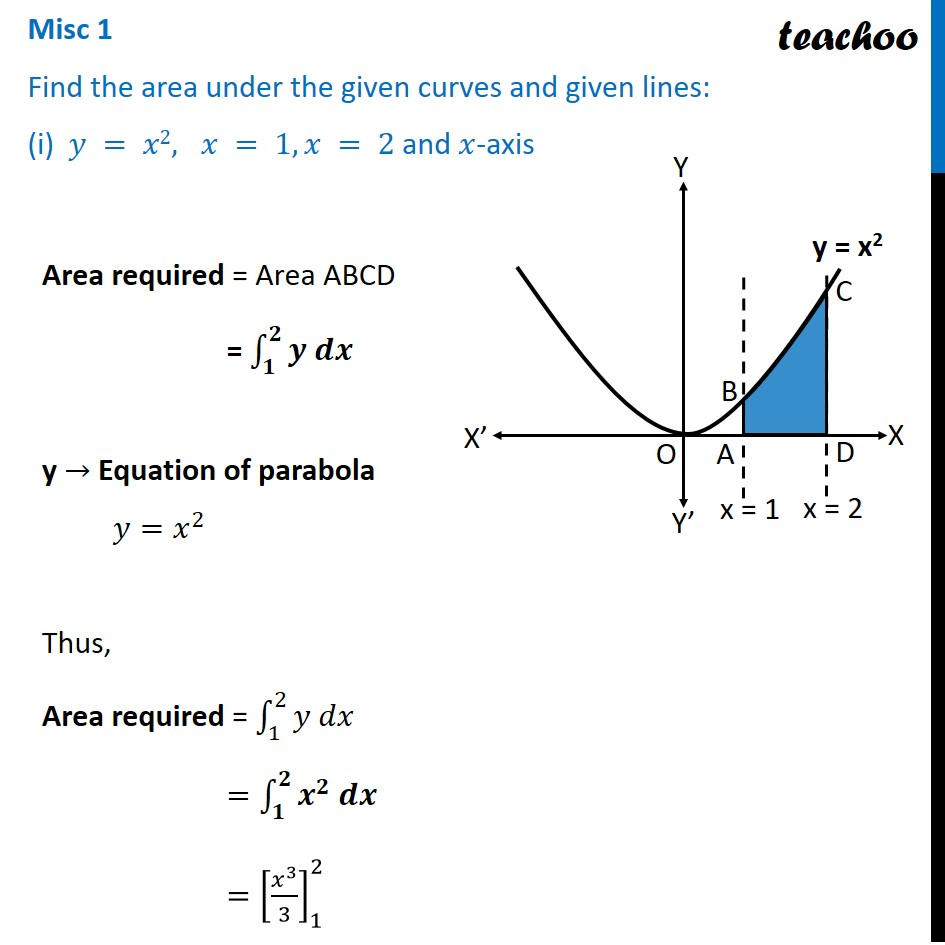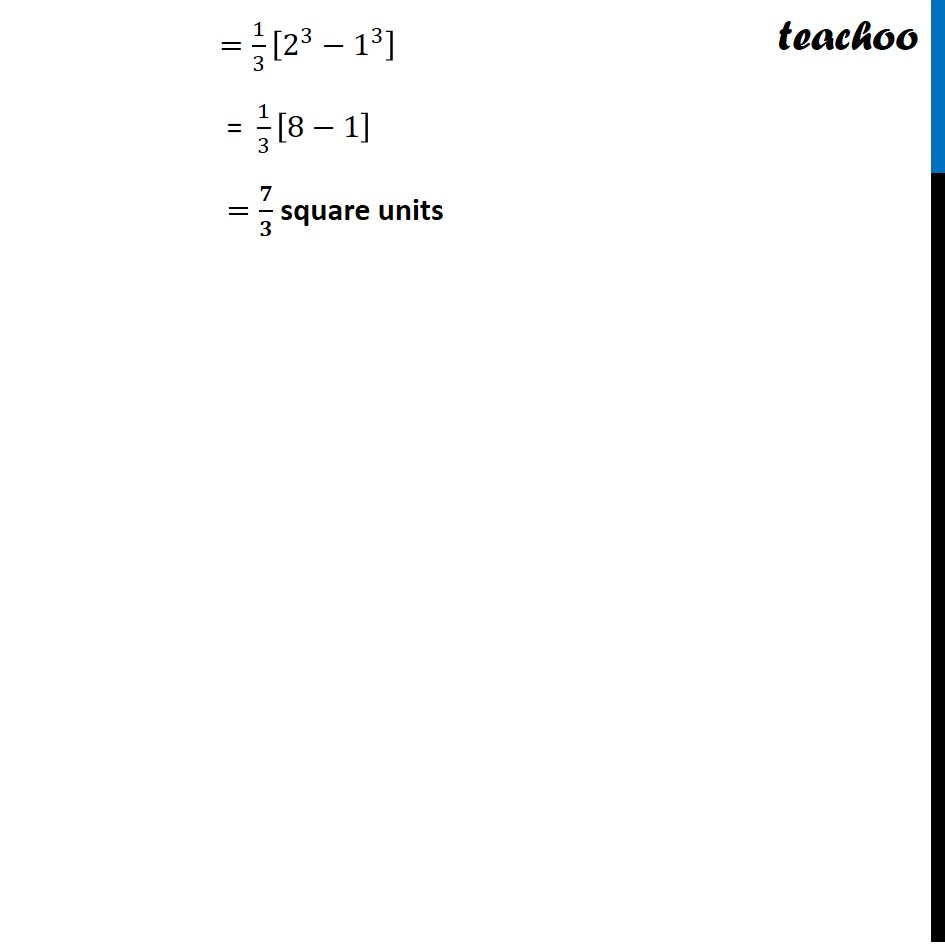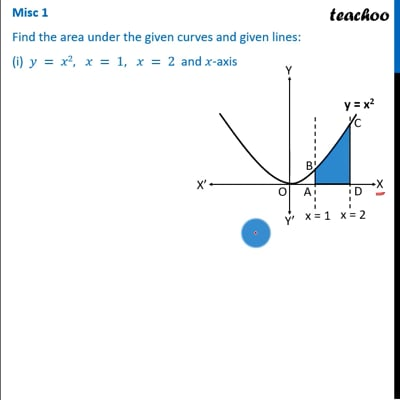Area bounded by curve and horizontal or vertical line

Chapter 8 Class 12 Application of Integrals
Concept wiseThis video is only available for Teachoo black users

Introducing your new favourite teacher - Teachoo Black, at only ₹83 per month

### Transcript

Misc 1 Find the area under the given curves and given lines: (i) 𝑦 = 𝑥2, 𝑥 = 1, 𝑥 = 2 and 𝑥-axisArea required = Area ABCD = ∫_𝟏^𝟐▒〖𝒚 𝒅𝒙〗 y → Equation of parabola 𝑦=𝑥^2 Thus, Area required = ∫_1^2▒〖𝑦 𝑑𝑥〗 =∫_𝟏^𝟐▒〖𝒙^𝟐 𝒅𝒙〗 =[𝑥^3/3]_1^2 =1/3 [2^3−1^3 ] = 1/3 [8−1] =𝟕/𝟑 square units